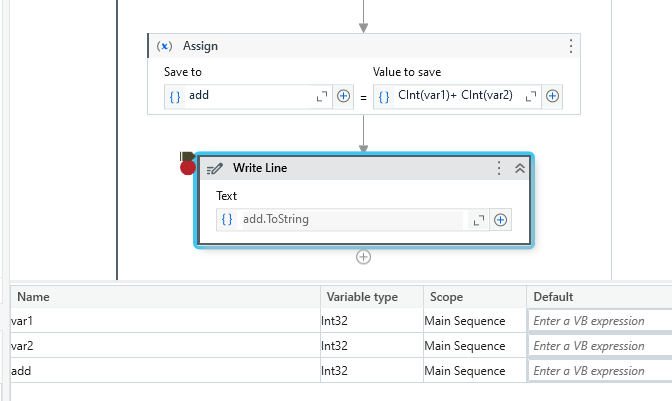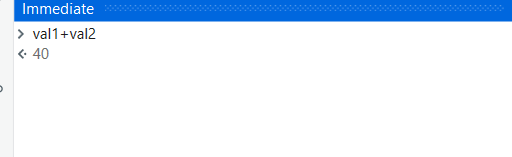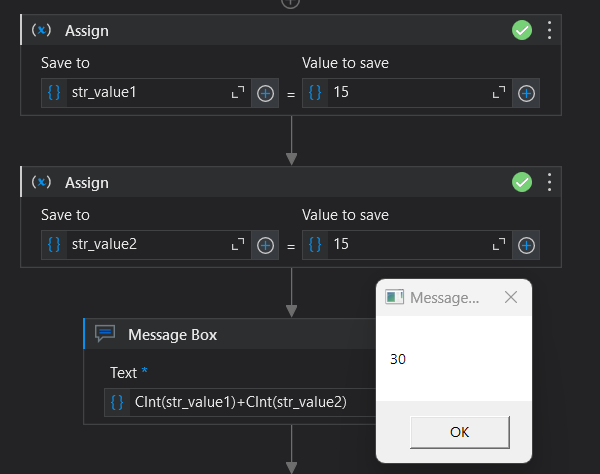Hi,

I tried to do addition using UI path Studio but after using below formula not getting proper answer.

1:- (Int_Number1+Int_Number2).ToString

Can any one help me on this.

getting out put same as what i value enter…just line

If i enter 15+15 then its give me out put is 1515.

You can try:
String.Concat(var1,var2) to get your desired result.
String.Join method is used to concatenate the elements of an array, using the specified separator between each element.

Hope it helps
Usha

Hi,

you’re trying to add two numbers and convert the result to a string in UiPath, but you’re getting unexpected results because you’re concatenating the numbers as strings instead of performing numerical addition first. To correctly add two numbers and then convert the result to a string, you should use the following expression:

(Int_Number1 + Int_Number2).ToString()

Make sure that `Int_Number1` and `Int_Number2` are variables or expressions that hold numeric values. The `+` operator should perform the addition, and then `.ToString()` will convert the result to a string.

Try like this

``````
Val1="15"
Val2="15"
Result=CInt(Val1)+CInt(Val2)

or

Val1=15
Val2=15
Result=Val1+Val2
``````

Hope this helps!!

It actually recognize the type of value you have given.
If you give 15+15 then UiPath takes it is an integer then gives 30
if you give “15”+“15” then it takes as string then gives 1515.

Hope it helps.

@Kamalesh_Chunekar
after adding don’t convert to string
assign to int variable

int_result=Int_Number1+Int_Number2

Hope it Helps!!!

its not working i tried this one also

it working great… thanks

@Kamalesh_Chunekar

You can also try this
cint(Int_Number1) + cint(Int_Number2)Try like this by changing it to integer

``````- Assign -> Output = (Cint(Int_Number1)+Cint(Int_Number2)).toString
``````

Use the above one which addition the two.

Check the below workflow for better understanding.

Hope it helps!!

ohh i thought it was not your requirement

and deleted the post
ok
Happy automation

1 Like

String.Concat(var1,var2) to get your desired result.
String.Join method is used to concatenate the elements of an array, using the specified separator between each element.

1 Like

resultYou can try this way alsoThis topic was automatically closed 3 days after the last reply. New replies are no longer allowed.